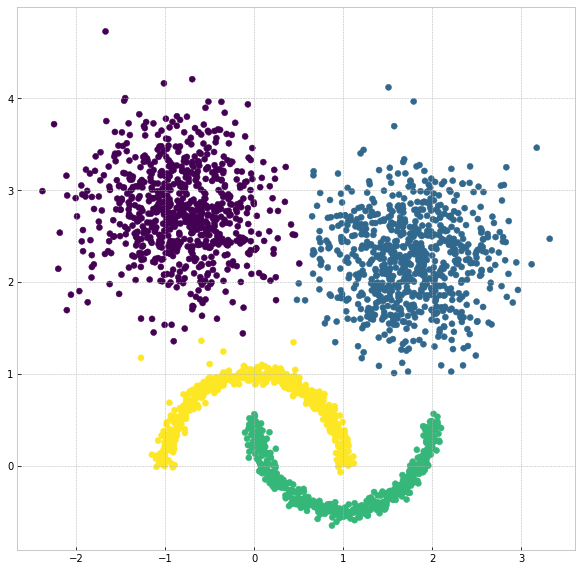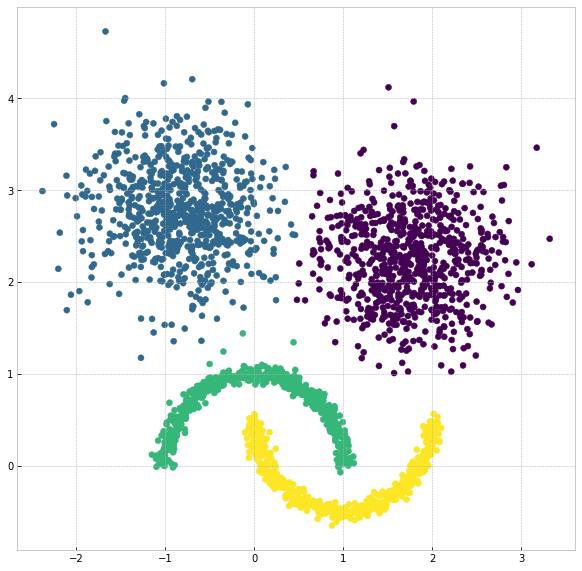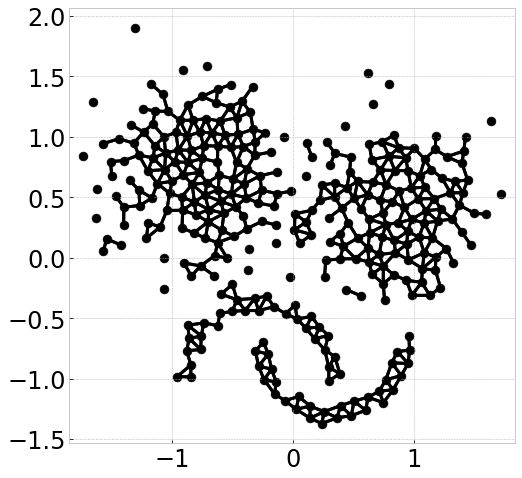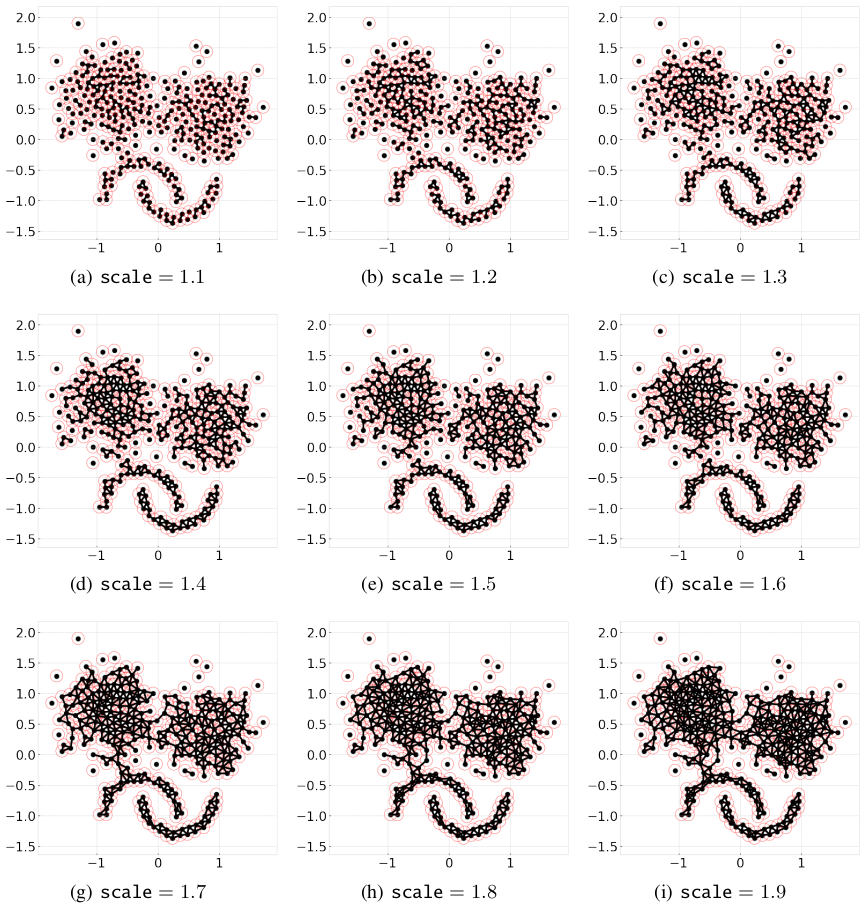# Clustering Tutorial

The CLASSIX with density method implements density clustering in an explicit way while the one with distance method implements density clustering in an implicit way. We will illustrate how to use them separately.

The examples here are demonstrated with a sample with a more complicated shape:

```import matplotlib.pyplot as plt
from sklearn import datasets
import numpy as np
random_state = 1
moons, _ = datasets.make_moons(n_samples=1000, noise=0.05, random_state=random_state)
blobs, _ = datasets.make_blobs(n_samples=1500, centers=[(-0.85,2.75), (1.75,2.25)], cluster_std=0.5, random_state=random_state)
X = np.vstack([blobs, moons])
```

Note

Setting radius lower, the more groups will form, and the groups tend to be separated instead of merging as clusters, and therefore runtime will increase.

## Density clustering

Instead of leaving the default option as the previous example, in this example, we can explicitly set a parameter `group_merging` to specify which merging strategy we would like to adopt. Also, we employ `sorting='pca'` or other choices such as ‘norm-orthant’ or ‘norm-mean’. In most cases, we recommend PCA sorting. Other available parameters include `radius` and `minPts`. The parameter of `radius` is a tolerance value dominating the aggregation phase, which immediately affects the merging phase. Usually, the thinner boundary between the clusters are, the lower `radius` is required. In addition, we would explain why we set minPts to 10 later. Also, we can output the log by setting verbose to 1, then it clearly shows how many clusters and the associated size we get:

```from classix import CLASSIX
clx = CLASSIX(sorting='pca', group_merging='density', radius=0.1, minPts=10)
clx.fit(X)
```

The output of the code is:

```CLASSIX(sorting='pca', radius=0.1, minPts=10, group_merging='density')
The 2500 data points were aggregated into 316 groups.
In total 16395 comparisons were required (6.56 comparisons per data point).
The 316 groups were merged into 47 clusters with the following sizes:
* cluster 0 : 717
* cluster 1 : 711
* cluster 2 : 500
* cluster 3 : 500
* cluster 4 : 10
* cluster 5 : 6
* cluster 6 : 3
* cluster 7 : 3
* cluster 8 : 3
* cluster 9 : 3
* cluster 10 : 3
* cluster 11 : 2
* cluster 12 : 2
* cluster 13 : 2
* cluster 14 : 2
* cluster 15 : 2
* cluster 16 : 1
* cluster 17 : 1
* cluster 18 : 1
* cluster 19 : 1
* cluster 20 : 1
* cluster 21 : 1
* cluster 22 : 1
* cluster 23 : 1
* cluster 24 : 1
* cluster 25 : 1
* cluster 26 : 1
* cluster 27 : 1
* cluster 28 : 1
* cluster 29 : 1
* cluster 30 : 1
* cluster 31 : 1
* cluster 32 : 1
* cluster 33 : 1
* cluster 34 : 1
* cluster 35 : 1
* cluster 36 : 1
* cluster 37 : 1
* cluster 38 : 1
* cluster 39 : 1
* cluster 40 : 1
* cluster 41 : 1
* cluster 42 : 1
* cluster 43 : 1
* cluster 44 : 1
* cluster 45 : 1
* cluster 46 : 1
As MinPts is 10, the number of clusters has been further reduced to 5.
Try the .explain() method to explain the clustering.
```

The reason why we set `minPts` to 10 is that we want the clusters with a size smaller than 10 to agglomerate to other big clusters which are partitioned significantly.

`minPts` is a parameter which we denote it as outliers threshold, and we will illustrate it in the section of `Outlier Detection`.

The visualization of clustering results is reasonable:

```plt.figure(figsize=(5,5))
plt.scatter(X[:,0], X[:,1], c=clx.labels_)
plt.show()
```## Distance clustering

The distance-based CLASSIX has the same steps as density-based CLASSIX except that the density comparison steps, in such a way distance-based CLASSIX does not require calculating the density, hence intuitively would be faster. By contrast, it just compares the pair of the clusters one at a time to determine if they should merge. Also, we propose a distance-based clustering exempted from calculating the density but with one more parameter for appropriate smoothing `scale`. By tuning the `scale`, we only calculate the distance between pairs of starting points and define the distance as the weights in the graph, and the distance that is smaller than \$texttt{scale}*radius\$ is assigned to 1 otherwise 0. The next step, similarly, is to find the connected components in the graph as clusters.

Similar to the previous example, we refer `group_merge` to ‘distance’, then adopt distance-based CLASSIX, the code is as below:

```clx= CLASSIX(sorting='pca', group_merging='distance', radius=0.1, minPts=4)
clx.fit(X)
```
```CLASSIX(sorting='pca', radius=0.1, method='distance')
The 2500 data points were aggregated into 316 groups.
In total 16395 comparisons were required (6.56 comparisons per data point).
The 316 groups were merged into 28 clusters with the following sizes:
* cluster 0 : 733
* cluster 1 : 730
* cluster 2 : 501
* cluster 3 : 500
* cluster 4 : 4
* cluster 5 : 4
* cluster 6 : 3
* cluster 7 : 2
* cluster 8 : 2
* cluster 9 : 2
* cluster 10 : 2
* cluster 11 : 1
* cluster 12 : 1
* cluster 13 : 1
* cluster 14 : 1
* cluster 15 : 1
* cluster 16 : 1
* cluster 17 : 1
* cluster 18 : 1
* cluster 19 : 1
* cluster 20 : 1
* cluster 21 : 1
* cluster 22 : 1
* cluster 23 : 1
* cluster 24 : 1
* cluster 25 : 1
* cluster 26 : 1
* cluster 27 : 1
As MinPts is 4, the number of clusters has been further reduced to 4.
Try the .explain() method to explain the clustering.
```

Visualize the result:

```plt.figure(figsize=(5,5))
plt.scatter(X[:,0], X[:,1], c=clx.labels_)
plt.show()
```Note

The density-based merging criterion usually results in slightly better clusters than the distance-based criterion, but the latter has a significant speed advantage.

## Visualize connecting edge

Now we use the same example to demonstrate how cluster are formed by computing starting points and edge connections. We can output the information by

```clx.visualize_linkage(scale=1.5, figsize=(8,8), labelsize=24, fmt='png')
```Note

The starting points can be interpreted as a reduced-density estimator of the data.

There is one more parameter that affects distance-based CLASSIX, that is `scale`. By simply adding the parameter `plot_boundary` and setting it to `True`, then we can obtain the starting points with their group boundary. The visualization of the connecting edge between starting points with varying `scale` is plotted as below:

```for scale in np.arange(1.1, 2, 0.1):
clx = CLASSIX(sorting='pca', radius=0.1, group_merging='distance', verbose=0)
clx.fit_transform(X)Considering a graph constructed by the starting points, as `scale` increases, the number of edges increases, therefore, the connected components area enlarges while the number of connected components decreases. Though in most cases, the scale setting is not necessary, when the small `radius` needed, adopting distance-based CLASSIX with an appropriate `scale` can greatly speed up the clustering application, such as image segmentation.